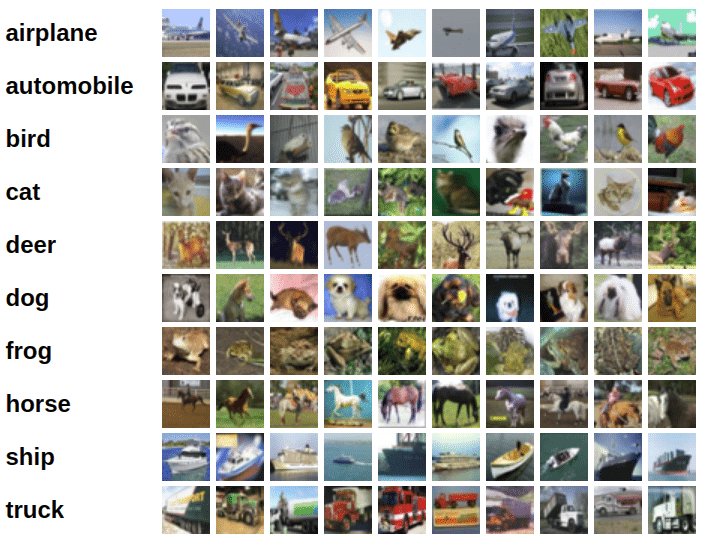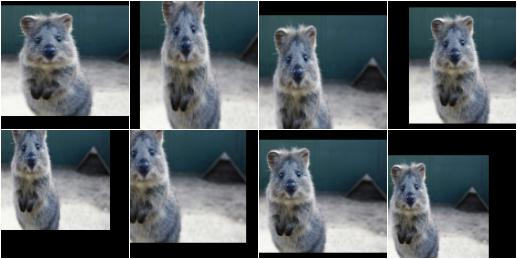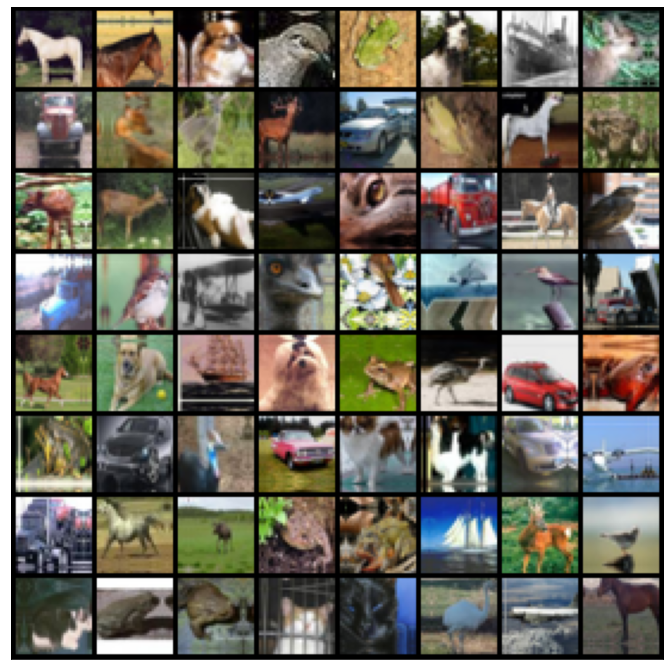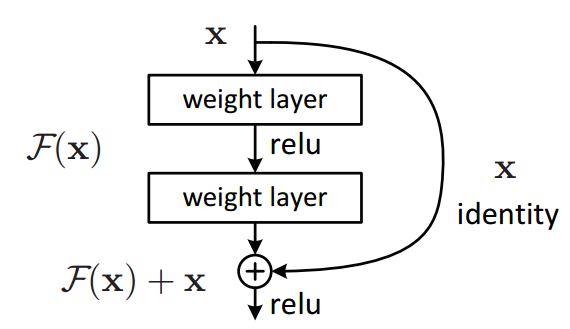## Classifying CIFAR10 images using ResNets, Regularization and Data Augmentation in PyTorch

A.K.A. Training an image classifier from scratch to over 90% accuracy in less than 5 minutes on a single GPU

#### Part 6 of "Deep Learning with Pytorch: Zero to GANs"

This tutorial series is a hands-on beginner-friendly introduction to deep learning using PyTorch, an open-source neural networks library. These tutorials take a practical and coding-focused approach. The best way to learn the material is to execute the code and experiment with it yourself. Check out the full series here:

In this tutorial, we'll use the following techniques to train a state-of-the-art model in less than 5 minutes to achieve over 90% accuracy in classifying images from the CIFAR10 dataset:

• Data normalization
• Data augmentation
• Residual connections
• Batch normalization
• Learning rate scheduling
• Weight Decay
• Gradient clipping
• Adam optimizer

#### How to run the code

This tutorial is an executable Jupyter notebook hosted on Jovian. You can run this tutorial and experiment with the code examples in a couple of ways: using free online resources (recommended) or on your computer.

##### Option 1: Running using free online resources (1-click, recommended)

The easiest way to start executing the code is to click the Run button at the top of this page and select Run on Colab. Google Colab is a free online platform for running Jupyter notebooks using Google's cloud infrastructure. You can also select "Run on Binder" or "Run on Kaggle" if you face issues running the notebook on Google Colab.

##### Option 2: Running on your computer locally

To run the code on your computer locally, you'll need to set up Python, download the notebook and install the required libraries. We recommend using the Conda distribution of Python. Click the Run button at the top of this page, select the Run Locally option, and follow the instructions.

#### Using a GPU for faster training

You can use a Graphics Processing Unit (GPU) to train your models faster if your execution platform is connected to a GPU manufactured by NVIDIA. Follow these instructions to use a GPU on the platform of your choice:

• Google Colab: Use the menu option "Runtime > Change Runtime Type" and select "GPU" from the "Hardware Accelerator" dropdown.
• Kaggle: In the "Settings" section of the sidebar, select "GPU" from the "Accelerator" dropdown. Use the button on the top-right to open the sidebar.
• Binder: Notebooks running on Binder cannot use a GPU, as the machines powering Binder aren't connected to any GPUs.
• Linux: If your laptop/desktop has an NVIDIA GPU (graphics card), make sure you have installed the NVIDIA CUDA drivers.
• Windows: If your laptop/desktop has an NVIDIA GPU (graphics card), make sure you have installed the NVIDIA CUDA drivers.
• macOS: macOS is not compatible with NVIDIA GPUs

If you do not have access to a GPU or aren't sure what it is, don't worry, you can execute all the code in this tutorial just fine without a GPU.

Let's begin by installing and importing the required libraries.

In :
``````# Uncomment and run the appropriate command for your operating system, if required
# No installation is reqiured on Google Colab / Kaggle notebooks

# Linux / Binder / Windows (No GPU)
# !pip install numpy matplotlib torch==1.7.0+cpu torchvision==0.8.1+cpu torchaudio==0.7.0 -f https://download.pytorch.org/whl/torch_stable.html

# Linux / Windows (GPU)
# pip install torch==1.7.1+cu110 torchvision==0.8.2+cu110 torchaudio==0.7.2 -f https://download.pytorch.org/whl/torch_stable.html

# MacOS (NO GPU)
# !pip install numpy matplotlib torch torchvision torchaudio``````
In :
``````import os
import torch
import torchvision
import tarfile
import torch.nn as nn
import numpy as np
import torch.nn.functional as F
from torchvision.datasets.utils import download_url
from torchvision.datasets import ImageFolder
from torch.utils.data import DataLoader
import torchvision.transforms as tt
from torch.utils.data import random_split
from torchvision.utils import make_grid
import matplotlib
import matplotlib.pyplot as plt
%matplotlib inline

matplotlib.rcParams['figure.facecolor'] = '#ffffff'``````
In :
``project_name='05b-cifar10-resnet'``

### Preparing the CIFAR10 Dataset

This notebook is an extension to the tutorial Image Classification using CNNs in PyTorch, where we trained a deep convolutional neural network to classify images from the CIFAR10 dataset with around 75% accuracy. Here are some images from the dataset:Let's begin by downloading the dataset and creating PyTorch datasets to load the data, just as we did in the previous tutorial.

In :
``````from torchvision.datasets.utils import download_url

# Dowload the dataset
dataset_url = "https://s3.amazonaws.com/fast-ai-imageclas/cifar10.tgz"
download_url(dataset_url, '.')

# Extract from archive
with tarfile.open('./cifar10.tgz', 'r:gz') as tar:
tar.extractall(path='./data')

# Look into the data directory
data_dir = './data/cifar10'
print(os.listdir(data_dir))
classes = os.listdir(data_dir + "/train")
print(classes)
``````
```Downloading https://s3.amazonaws.com/fast-ai-imageclas/cifar10.tgz to ./cifar10.tgz ```
``HBox(children=(FloatProgress(value=1.0, bar_style='info', max=1.0), HTML(value='')))``
``` ['train', 'test'] ['frog', 'airplane', 'truck', 'horse', 'dog', 'bird', 'ship', 'automobile', 'deer', 'cat'] ```

We can create training and validation datasets using the `ImageFolder` class from `torchvision`. In addition to the `ToTensor` transform, we'll also apply some other transforms to the images. There are a few important changes we'll make while creating PyTorch datasets for training and validation:

1. Use test set for validation: Instead of setting aside a fraction (e.g. 10%) of the data from the training set for validation, we'll simply use the test set as our validation set. This just gives a little more data to train with. In general, once you have picked the best model architecture & hypeparameters using a fixed validation set, it is a good idea to retrain the same model on the entire dataset just to give it a small final boost in performance.
2. Channel-wise data normalization: We will normalize the image tensors by subtracting the mean and dividing by the standard deviation across each channel. As a result, the mean of the data across each channel is 0, and standard deviation is 1. Normalizing the data prevents the values from any one channel from disproportionately affecting the losses and gradients while training, simply by having a higher or wider range of values that others.1. Randomized data augmentations: We will apply randomly chosen transformations while loading images from the training dataset. Specifically, we will pad each image by 4 pixels, and then take a random crop of size 32 x 32 pixels, and then flip the image horizontally with a 50% probability. Since the transformation will be applied randomly and dynamically each time a particular image is loaded, the model sees slightly different images in each epoch of training, which allows it generalize better.In :
``````# Data transforms (normalization & data augmentation)
stats = ((0.4914, 0.4822, 0.4465), (0.2023, 0.1994, 0.2010))
train_tfms = tt.Compose([tt.RandomCrop(32, padding=4, padding_mode='reflect'),
tt.RandomHorizontalFlip(),
# tt.RandomRotate
# tt.RandomResizedCrop(256, scale=(0.5,0.9), ratio=(1, 1)),
# tt.ColorJitter(brightness=0.1, contrast=0.1, saturation=0.1, hue=0.1),
tt.ToTensor(),
tt.Normalize(*stats,inplace=True)])
valid_tfms = tt.Compose([tt.ToTensor(), tt.Normalize(*stats)])
``````
In :
``````# PyTorch datasets
train_ds = ImageFolder(data_dir+'/train', train_tfms)
valid_ds = ImageFolder(data_dir+'/test', valid_tfms)``````

Next, we can create data loaders for retrieving images in batches. We'll use a relatively large batch size of 500 to utlize a larger portion of the GPU RAM. You can try reducing the batch size & restarting the kernel if you face an "out of memory" error.

In :
``batch_size = 400``
In :
``````# PyTorch data loaders
train_dl = DataLoader(train_ds, batch_size, shuffle=True, num_workers=3, pin_memory=True)
valid_dl = DataLoader(valid_ds, batch_size*2, num_workers=3, pin_memory=True)``````

Let's take a look at some sample images from the training dataloader. To display the images, we'll need to denormalize the pixels values to bring them back into the range `(0,1)`.

In :
``````def denormalize(images, means, stds):
means = torch.tensor(means).reshape(1, 3, 1, 1)
stds = torch.tensor(stds).reshape(1, 3, 1, 1)
return images * stds + means

def show_batch(dl):
for images, labels in dl:
fig, ax = plt.subplots(figsize=(12, 12))
ax.set_xticks([]); ax.set_yticks([])
denorm_images = denormalize(images, *stats)
ax.imshow(make_grid(denorm_images[:64], nrow=8).permute(1, 2, 0).clamp(0,1))
break``````
In :
``show_batch(train_dl)``The colors seem out of place because of the normalization. Note that normalization is also applied during inference. If you look closely, you can see the cropping and reflection padding in some of the images. Horizontal flip is a bit difficult to detect from visual inspection.

### Using a GPU

To seamlessly use a GPU, if one is available, we define a couple of helper functions (`get_default_device` & `to_device`) and a helper class `DeviceDataLoader` to move our model & data to the GPU as required. These are described in more detail in a previous tutorial.

In :
``````def get_default_device():
"""Pick GPU if available, else CPU"""
if torch.cuda.is_available():
return torch.device('cuda')
else:
return torch.device('cpu')

def to_device(data, device):
"""Move tensor(s) to chosen device"""
if isinstance(data, (list,tuple)):
return [to_device(x, device) for x in data]
return data.to(device, non_blocking=True)

class DeviceDataLoader():
"""Wrap a dataloader to move data to a device"""
def __init__(self, dl, device):
self.dl = dl
self.device = device

def __iter__(self):
"""Yield a batch of data after moving it to device"""
for b in self.dl:
yield to_device(b, self.device)

def __len__(self):
"""Number of batches"""
return len(self.dl)``````

Based on where you're running this notebook, your default device could be a CPU (`torch.device('cpu')`) or a GPU (`torch.device('cuda')`)

In :
``````device = get_default_device()
device
``````
Out:
``device(type='cuda')``

We can now wrap our training and validation data loaders using `DeviceDataLoader` for automatically transferring batches of data to the GPU (if available).

In :
``````train_dl = DeviceDataLoader(train_dl, device)
valid_dl = DeviceDataLoader(valid_dl, device)
``````

### Model with Residual Blocks and Batch Normalization

One of the key changes to our CNN model this time is the addition of the resudial block, which adds the original input back to the output feature map obtained by passing the input through one or more convolutional layers.Here is a very simple Residual block:

In :
``````class SimpleResidualBlock(nn.Module):
def __init__(self):
super().__init__()
self.conv1 = nn.Conv2d(in_channels=3, out_channels=3, kernel_size=3, stride=1, padding=1)
self.relu1 = nn.ReLU()
self.conv2 = nn.Conv2d(in_channels=3, out_channels=3, kernel_size=3, stride=1, padding=1)
self.relu2 = nn.ReLU()

def forward(self, x):
out = self.conv1(x)
out = self.relu1(out)
out = self.conv2(out)
return self.relu2(out) + x # ReLU can be applied before or after adding the input``````
In :
``````simple_resnet = to_device(SimpleResidualBlock(), device)

for images, labels in train_dl:
out = simple_resnet(images)
print(out.shape)
break

del simple_resnet, images, labels
torch.cuda.empty_cache()
``````
```torch.Size([400, 3, 32, 32]) ```

This seeming small change produces a drastic improvement in the performance of the model. Also, after each convolutional layer, we'll add a batch normalization layer, which normalizes the outputs of the previous layer.

Go through the following blog posts to learn more:

We will use the ResNet9 architecture, as described in this blog series :In :
``````def accuracy(outputs, labels):
_, preds = torch.max(outputs, dim=1)
return torch.tensor(torch.sum(preds == labels).item() / len(preds))

class ImageClassificationBase(nn.Module):
def training_step(self, batch):
images, labels = batch
out = self(images)                  # Generate predictions
loss = F.cross_entropy(out, labels) # Calculate loss
return loss

def validation_step(self, batch):
images, labels = batch
out = self(images)                    # Generate predictions
loss = F.cross_entropy(out, labels)   # Calculate loss
acc = accuracy(out, labels)           # Calculate accuracy
return {'val_loss': loss.detach(), 'val_acc': acc}

def validation_epoch_end(self, outputs):
batch_losses = [x['val_loss'] for x in outputs]
epoch_loss = torch.stack(batch_losses).mean()   # Combine losses
batch_accs = [x['val_acc'] for x in outputs]
epoch_acc = torch.stack(batch_accs).mean()      # Combine accuracies
return {'val_loss': epoch_loss.item(), 'val_acc': epoch_acc.item()}

def epoch_end(self, epoch, result):
print("Epoch [{}], last_lr: {:.5f}, train_loss: {:.4f}, val_loss: {:.4f}, val_acc: {:.4f}".format(
epoch, result['lrs'][-1], result['train_loss'], result['val_loss'], result['val_acc']))``````
In :
``````def conv_block(in_channels, out_channels, pool=False):
layers = [nn.Conv2d(in_channels, out_channels, kernel_size=3, padding=1),
nn.BatchNorm2d(out_channels),
nn.ReLU(inplace=True)]
if pool: layers.append(nn.MaxPool2d(2))
return nn.Sequential(*layers)

class ResNet9(ImageClassificationBase):
def __init__(self, in_channels, num_classes):
super().__init__()

self.conv1 = conv_block(in_channels, 64)
self.conv2 = conv_block(64, 128, pool=True)
self.res1 = nn.Sequential(conv_block(128, 128), conv_block(128, 128))

self.conv3 = conv_block(128, 256, pool=True)
self.conv4 = conv_block(256, 512, pool=True)
self.res2 = nn.Sequential(conv_block(512, 512), conv_block(512, 512))

self.classifier = nn.Sequential(nn.MaxPool2d(4),
nn.Flatten(),
nn.Dropout(0.2),
nn.Linear(512, num_classes))

def forward(self, xb):
out = self.conv1(xb)
out = self.conv2(out)
out = self.res1(out) + out
out = self.conv3(out)
out = self.conv4(out)
out = self.res2(out) + out
out = self.classifier(out)
return out``````
In :
``````model = to_device(ResNet9(3, 10), device)
model
``````
Out:
``````ResNet9(
(conv1): Sequential(
(0): Conv2d(3, 64, kernel_size=(3, 3), stride=(1, 1), padding=(1, 1))
(1): BatchNorm2d(64, eps=1e-05, momentum=0.1, affine=True, track_running_stats=True)
(2): ReLU(inplace=True)
)
(conv2): Sequential(
(0): Conv2d(64, 128, kernel_size=(3, 3), stride=(1, 1), padding=(1, 1))
(1): BatchNorm2d(128, eps=1e-05, momentum=0.1, affine=True, track_running_stats=True)
(2): ReLU(inplace=True)
(3): MaxPool2d(kernel_size=2, stride=2, padding=0, dilation=1, ceil_mode=False)
)
(res1): Sequential(
(0): Sequential(
(0): Conv2d(128, 128, kernel_size=(3, 3), stride=(1, 1), padding=(1, 1))
(1): BatchNorm2d(128, eps=1e-05, momentum=0.1, affine=True, track_running_stats=True)
(2): ReLU(inplace=True)
)
(1): Sequential(
(0): Conv2d(128, 128, kernel_size=(3, 3), stride=(1, 1), padding=(1, 1))
(1): BatchNorm2d(128, eps=1e-05, momentum=0.1, affine=True, track_running_stats=True)
(2): ReLU(inplace=True)
)
)
(conv3): Sequential(
(0): Conv2d(128, 256, kernel_size=(3, 3), stride=(1, 1), padding=(1, 1))
(1): BatchNorm2d(256, eps=1e-05, momentum=0.1, affine=True, track_running_stats=True)
(2): ReLU(inplace=True)
(3): MaxPool2d(kernel_size=2, stride=2, padding=0, dilation=1, ceil_mode=False)
)
(conv4): Sequential(
(0): Conv2d(256, 512, kernel_size=(3, 3), stride=(1, 1), padding=(1, 1))
(1): BatchNorm2d(512, eps=1e-05, momentum=0.1, affine=True, track_running_stats=True)
(2): ReLU(inplace=True)
(3): MaxPool2d(kernel_size=2, stride=2, padding=0, dilation=1, ceil_mode=False)
)
(res2): Sequential(
(0): Sequential(
(0): Conv2d(512, 512, kernel_size=(3, 3), stride=(1, 1), padding=(1, 1))
(1): BatchNorm2d(512, eps=1e-05, momentum=0.1, affine=True, track_running_stats=True)
(2): ReLU(inplace=True)
)
(1): Sequential(
(0): Conv2d(512, 512, kernel_size=(3, 3), stride=(1, 1), padding=(1, 1))
(1): BatchNorm2d(512, eps=1e-05, momentum=0.1, affine=True, track_running_stats=True)
(2): ReLU(inplace=True)
)
)
(classifier): Sequential(
(0): MaxPool2d(kernel_size=4, stride=4, padding=0, dilation=1, ceil_mode=False)
(1): Flatten(start_dim=1, end_dim=-1)
(2): Dropout(p=0.2, inplace=False)
(3): Linear(in_features=512, out_features=10, bias=True)
)
)``````

### Training the model

Before we train the model, we're going to make a bunch of small but important improvements to our `fit` function:

• Learning rate scheduling: Instead of using a fixed learning rate, we will use a learning rate scheduler, which will change the learning rate after every batch of training. There are many strategies for varying the learning rate during training, and the one we'll use is called the "One Cycle Learning Rate Policy", which involves starting with a low learning rate, gradually increasing it batch-by-batch to a high learning rate for about 30% of epochs, then gradually decreasing it to a very low value for the remaining epochs. Learn more: https://sgugger.github.io/the-1cycle-policy.html

• Weight decay: We also use weight decay, which is yet another regularization technique which prevents the weights from becoming too large by adding an additional term to the loss function.Learn more: https://towardsdatascience.com/this-thing-called-weight-decay-a7cd4bcfccab

• Gradient clipping: Apart from the layer weights and outputs, it also helpful to limit the values of gradients to a small range to prevent undesirable changes in parameters due to large gradient values. This simple yet effective technique is called gradient clipping. Learn more: https://towardsdatascience.com/what-is-gradient-clipping-b8e815cdfb48

Let's define a `fit_one_cycle` function to incorporate these changes. We'll also record the learning rate used for each batch.

In :
``````@torch.no_grad()
def evaluate(model, val_loader):
model.eval()
outputs = [model.validation_step(batch) for batch in val_loader]
return model.validation_epoch_end(outputs)

def get_lr(optimizer):
for param_group in optimizer.param_groups:
return param_group['lr']

def fit_one_cycle(epochs, max_lr, model, train_loader, val_loader,
weight_decay=0, grad_clip=None, opt_func=torch.optim.SGD):
torch.cuda.empty_cache()
history = []

# Set up cutom optimizer with weight decay
optimizer = opt_func(model.parameters(), max_lr, weight_decay=weight_decay)
# Set up one-cycle learning rate scheduler
sched = torch.optim.lr_scheduler.OneCycleLR(optimizer, max_lr, epochs=epochs,
steps_per_epoch=len(train_loader))

for epoch in range(epochs):
# Training Phase
model.train()
train_losses = []
lrs = []
for batch in train_loader:
loss = model.training_step(batch)
train_losses.append(loss)
loss.backward()

# Gradient clipping
if grad_clip:
nn.utils.clip_grad_value_(model.parameters(), grad_clip)

optimizer.step()
optimizer.zero_grad()

# Record & update learning rate
lrs.append(get_lr(optimizer))
sched.step()

# Validation phase
result = evaluate(model, val_loader)
result['train_loss'] = torch.stack(train_losses).mean().item()
result['lrs'] = lrs
model.epoch_end(epoch, result)
history.append(result)
return history``````
In :
``````history = [evaluate(model, valid_dl)]
history
``````
Out:
``[{'val_acc': 0.1251922994852066, 'val_loss': 2.303567886352539}]``

We're now ready to train our model. Instead of SGD (stochastic gradient descent), we'll use the Adam optimizer which uses techniques like momentum and adaptive learning rates for faster training. You can learn more about optimizers here: https://ruder.io/optimizing-gradient-descent/index.html

In :
``````epochs = 8
max_lr = 0.01
grad_clip = 0.1
weight_decay = 1e-4
opt_func = torch.optim.Adam
``````
In :
``````%%time
history += fit_one_cycle(epochs, max_lr, model, train_dl, valid_dl,
grad_clip=grad_clip,
weight_decay=weight_decay,
opt_func=opt_func)
``````
```Epoch , last_lr: 0.00393, train_loss: 1.5018, val_loss: 2.3667, val_acc: 0.4839 Epoch , last_lr: 0.00935, train_loss: 1.0881, val_loss: 1.8056, val_acc: 0.5457 Epoch , last_lr: 0.00972, train_loss: 0.8391, val_loss: 1.1632, val_acc: 0.6179 Epoch , last_lr: 0.00812, train_loss: 0.6418, val_loss: 0.7527, val_acc: 0.7377 Epoch , last_lr: 0.00556, train_loss: 0.5059, val_loss: 0.5230, val_acc: 0.8203 Epoch , last_lr: 0.00283, train_loss: 0.4056, val_loss: 0.4061, val_acc: 0.8620 Epoch , last_lr: 0.00077, train_loss: 0.2901, val_loss: 0.3132, val_acc: 0.8925 Epoch , last_lr: 0.00000, train_loss: 0.2227, val_loss: 0.2730, val_acc: 0.9055 CPU times: user 37.5 s, sys: 16 s, total: 53.5 s Wall time: 4min 24s ```
In :
``train_time='4:24'``

Our model trained to over 90% accuracy in under 5 minutes! Try playing around with the data augmentations, network architecture & hyperparameters to achive the following results:

1. 94% accuracy in under 10 minutes (easy)
2. 90% accuracy in under 2.5 minutes (intermediate)
3. 94% accuracy in under 5 minutes (hard)

Let's plot the valdation set accuracies to study how the model improves over time.

In :
``````def plot_accuracies(history):
accuracies = [x['val_acc'] for x in history]
plt.plot(accuracies, '-x')
plt.xlabel('epoch')
plt.ylabel('accuracy')
plt.title('Accuracy vs. No. of epochs');``````
In :
``plot_accuracies(history)``